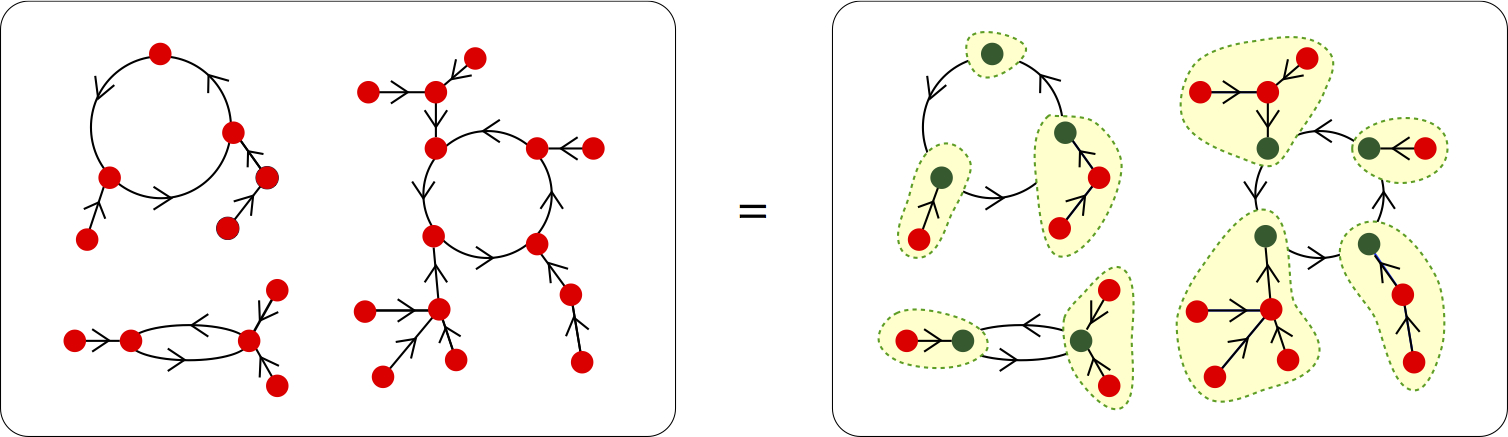# Combinatorial Species

Following a slow (and forced) evolution in the history of Mathematics, the modern notion of function (due to Dirichlet, 1837) has been made independent of any actual description format. This simple idea has opened up the possibility of considering spaces of functions, and operators on these. A similar process has led André Joyal  to introduce in combinatorics the notion of  “Species”,  to free the description of combinatorial structures from a specific format. This theory serves as an elegant explanation for the surprising power of generating function uses for the solution of structure enumeration; but its true originality lies in the fact that it naturally takes into account the action of permutation groups on the structures considered.

During the last decades considerable progress has been made in clarifying and strengthening the foundations of enumerative combinatorics. A number of useful theories have emerged to explain the use of algebraic techniques in combinatorics. Among others, let us just mention Rota’s work on Möbius Inversion and Umbral Calculus, the theory of Binomial Posets of Stanley, and the manifold combinatorial applications of Hopf Algebras. Many authors, such as Goulden and Jackson, or Wilf, have made clear the importance of having good general methods to solve problems of enumeration. In particular Flajolet, Knuth, and Schützenberger have given deep reasons related to theoretical computer science. In addition, during this same period, Combinatorics has been greatly enriched by its large and varied interactions with Algebra and Theoretical Physics.

The combinatorial theory of species is set in this general undertaking. It  provides a unified understanding of the use of generating series for both labeled and unlabelled structures, as well as a tool for the specification and analysis of these structures. Of particular importance is its capacity to transform recursive definitions of (tree-like) structures into functional or differential equations, and conversely. Encompassing the description of structures together with permutation group actions, the theory of species conciliates the calculus of generating series and functional equations with Pólya theory. Formally, all this is achieved by considering functors from the category of finite sets and bijections to itself, together with an algebraic framework on these functors. However, the actual use of categorical notions is quite lite.A pictorial proof in the context of species

Explicitly, a species of structures  $F$ is simply a pair of rules: one associating to each finite set $U$, a finite set $F[U]$; and another associating to each biijection $\sigma:U\rightarrow V$, a bijection $F[\sigma]:F[U]\rightarrow F{V}$. Members of the set $F[U]$, are the $F$-structures on the underlying set $U$. The functoriality condition corresponds to the requirement that the association $\sigma\mapsto F[\sigma]$ be consistent with composition of bijections. In this way the concept of species of structures puts as much emphasis on isomorphisms as on the structures themselves.

Species of structures can be combined to form new species by using set theoretical constructions. There results a variety of combinatorial operations on species, including addition, multiplication, substitution, derivation, etc, which extend the familiar calculus of formal power series. Indeed to each species of structures, one can associate various formal power series designed to treat enumeration problems of a specific kind (labeled, unlabelled, asymmetric, weighted, etc.). Of key importance is the fact that these associated series are “compatible” with operations on species. Hence each (algebraic, functional or differential) identity between species implies identities between their associated series. This is in the spirit of Euler’s method of generating series. The power of the Theory of Species is best appreciated when one sees how one simple argument, proving identities between species, automatically implies both classical combinatorial identities and Pólya-like formulas (as well as symmetric function identities and more).

For more on all this, see